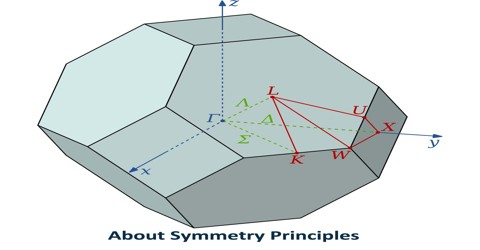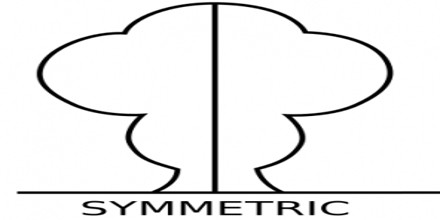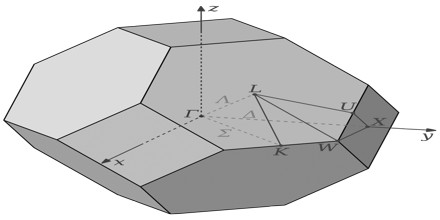PhysicsDefinition of Symmetry

In physics, Symmetry the properties of particles such as atoms and molecules remain unchanged after being subjected to a variety of symmetry transformations or “operations.”

A family of particular transformations may be continuous, such as rotation of a circle or discrete, e.g., reflection of a bilaterally symmetric figure, or rotation of a regular polygon. Continuous and discrete transformations give rise to corresponding types of symmetries. Continuous symmetries can be described by Lie groups while discrete symmetries are described by finite groups. The two outstanding theoretical achievements of the 20th century, relativity and quantum mechanics, involve notions of symmetry in a fundamental way.Arguably the most important example of a symmetry in physics is that the speed of light has the same value in all frames of reference, which is known in mathematical terms as Poincaré group, the symmetry group of special relativity. Another important example is the invariance of the form of physical laws under arbitrary differentiable coordinate transformations, which is an important idea in general relativity.

Symmetries may be broadly classified as global or local. A global symmetry is one that holds at all points of spacetime, whereas a local symmetry is one that has a different symmetry transformation at different points of spacetime; specifically a local symmetry transformation is parameterised by the spacetime co-ordinates. Local symmetries play an important role in physics as they form the basis for gauge theories.

Symmetry Principles

Symmetry Principles starts with the discussion of the concept of causal relation in physical systems, whereby certain correlations exist between states of cause subsystems and states of effect subsystems, correlations resulting from the fact that states of subsystems are determined by the states of the whole system. Then the concepts of equivalence relation and equivalence class are presented. Scientific laws as expressions of causal relations. Such laws must ignore certain aspects of states of physical systems. From the equivalence principle is derived the symmetry principle: A symmetry transformation of the cause is also a symmetry transformation of the effect. Equivalently: The symmetry group of the cause is a subgroup of the symmetry group of the effect. Alternatively: The effect is at least as symmetric as the cause.Symmetries allow people—and scientists—to discern order in nature. In physics, it has long been understood that symmetries are closely connected to conservation laws. Three of the most familiar conservation laws are the conservation of energy, the conservation of momentum, and the conservation of angular momentum.

Conservation of energy is a consequence of the fact that the laws of nature do not change with time. For example, in Newton’s law of gravitation, one could imagine that GN, the gravitational constant, depended on time. In this case, energy would not be conserved. From experimental searches for violations of energy conservation, one can set strong limits on any such time variation, astronomical observations provide stronger constraints. This principle is quite broad and applies in quantum mechanics as well as classical mechanics. Physicists sometimes call this symmetry—that there is no special time—the “homogeneity of time.”

Conservation of momentum is a consequence of the fact that there is no special place. If one describes the world with Cartesian coordinates, the laws of nature don’t care what one takes to be the origin. This symmetry is called “translation invariance,” or the homogeneity of space.

Conservation of angular momentum is related to a familiar symmetry of daily life: the laws of nature are invariant under rotations. For example, not only does it not matter how we choose the origin or our coordinate system, but it doesn’t matter how we choose to orient the axes.

The symmetries of time and space translation, and rotations, are called continuous symmetries because one can translate the coordinate axes by any arbitrary amount, and one can rotate through any angle. Another class of symmetries is called discrete symmetries. Another way to describe this symmetry is as symmetry between left and right.

An important type of physical theory based on local symmetries is called a gauge theory and the symmetries natural to such a theory are called gauge symmetries. Gauge symmetries in the Standard model, used to describe three of the fundamental interactions, are based on the SU(3) × SU(2) × U(1) group. Roughly speaking, the symmetries of the SU(3) group describe the strong force, the SU(2) group describes the weak interaction and the U(1) group describes the electromagnetic force.

Also, the reduction by symmetry of the energy functional under the action by a group and spontaneous symmetry breaking of transformations of symmetric groups appear to elucidate topics in particle physics, for example, the unification of electromagnetism and the weak force in physical cosmology.